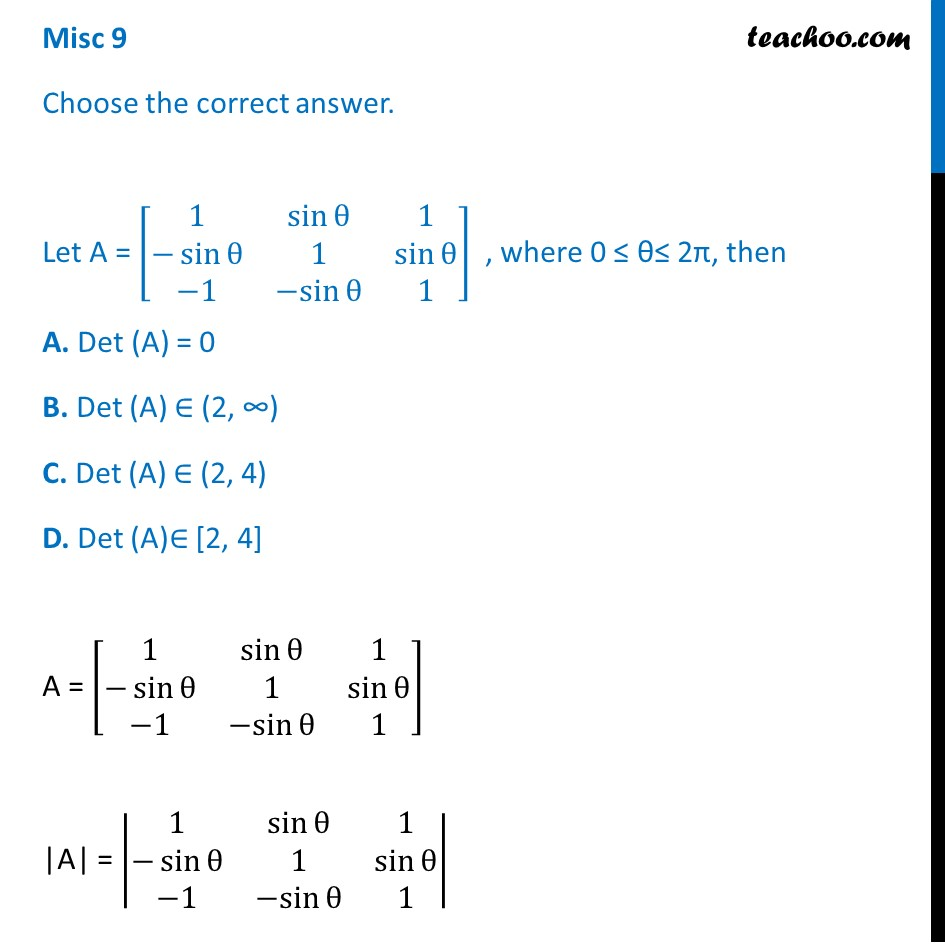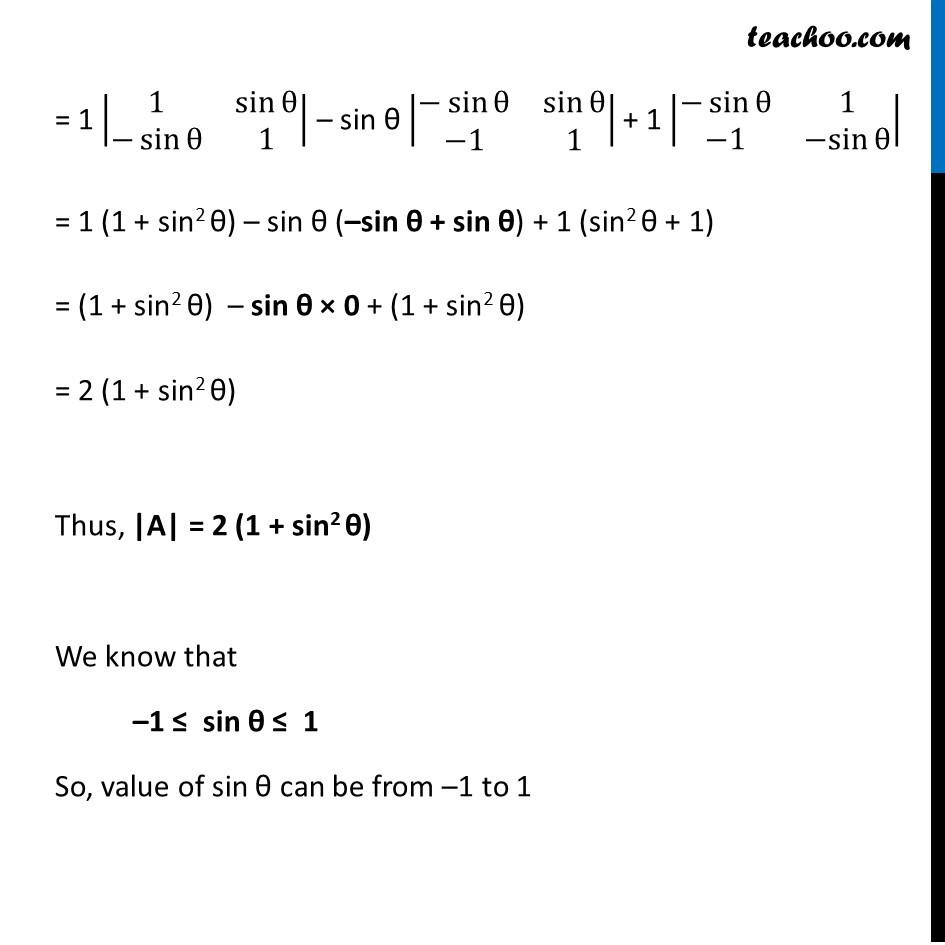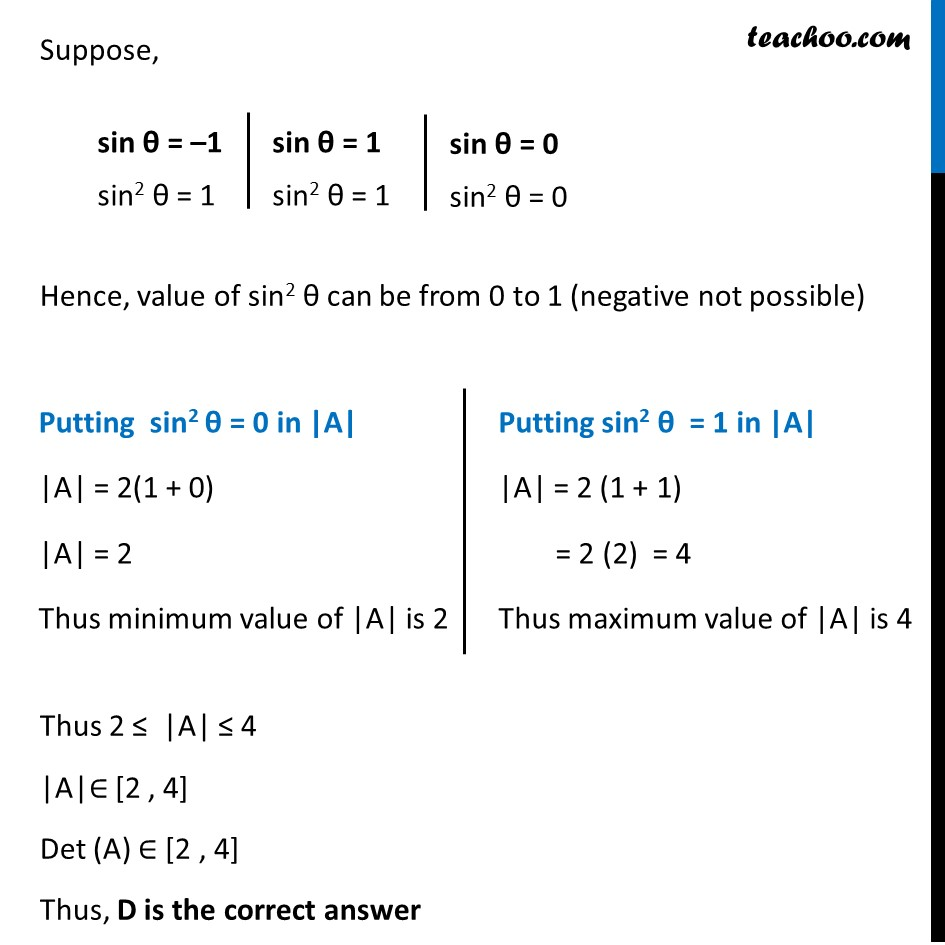Miscellaneous

Chapter 4 Class 12 Determinants
Serial order wiseLearn in your speed, with individual attention - Teachoo Maths 1-on-1 Class

### Transcript

Misc 9 Choose the correct answer. Let A = [■8(1&sin⁡θ&1@−sin⁡θ&1&sin⁡θ@−1&〖−sin〗⁡θ&1)] , where 0 ≤ θ≤ 2π, then A. Det (A) = 0 B. Det (A) ∈ (2, ∞) C. Det (A) ∈ (2, 4) D. Det (A)∈ [2, 4] A = [■8(1&sin⁡θ&1@−sin⁡θ&1&sin⁡θ@−1&〖−sin〗⁡θ&1)] |A| = |■8(1&sin⁡θ&1@−sin⁡θ&1&sin⁡θ@−1&〖−sin〗⁡θ&1)| = 1 |■8(1&sin⁡θ@−sin⁡θ&1)| – sin θ |■8(−sin⁡θ&sin⁡θ@−1&1)| + 1 |■8(−sin⁡θ&1@−1&〖−sin〗⁡θ )| = 1 (1 + sin2 θ) – sin θ (–sin θ + sin θ) + 1 (sin2 θ + 1) = (1 + sin2 θ) – sin θ × 0 + (1 + sin2 θ) = 2 (1 + sin2 θ) Thus, |A| = 2 (1 + sin2 θ) We know that –1 ≤ sin θ ≤ 1 So, value of sin θ can be from –1 to 1 Suppose, Hence, value of sin2 θ can be from 0 to 1 (negative not possible) Thus 2 ≤ |A| ≤ 4 |A|∈ [2 , 4] Det (A) ∈ [2 , 4] Thus, D is the correct answer Putting sin2 θ = 0 in |A| |A| = 2(1 + 0) |A| = 2 Thus minimum value of |A| is 2 Putting sin2 θ = 1 in |A| |A| = 2 (1 + 1) = 2 (2) = 4 Thus maximum value of |A| is 4